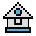Home

# DRAW Statement

#### Purpose:

To draw a figure.

#### Syntax:

`DRAW string expression`

The DRAW statement combines most of the capabilities of the other graphics statements into an object definition language called Graphics Macro Language (GML). A GML command is a single character within a string, optionally followed by one or more arguments.

The DRAW statement is valid only in graphics mode.

Movement Commands:

Each of the following movement commands begins movement from the current graphics position. This is usually the coordinate of the last graphics point plotted with another GML command, LINE, or PSET. The current position defaults to the center of the screen (160,100 in medium resolution; 320,100 in high resolution) when a program is run. Movement commands move for a distance of scale factor *n, where the default for n is 1; thus, they move one point if n is omitted and the default scale factor is used.

 Command Moves Un up Dn down Ln left Rn right En diagonally up and right Fn diagonally down and right Gn diagonally down and left Hn diagonally up and left

This command moves as specified by the following argument:

 Mx, y Move absolute or relative. If x is preceded by a + or -, x and y are added to the current graphics position, and connected to the current position by a line. Otherwise, a line is drawn to point x, y from the current position.

The following prefix commands may precede any of the above movement commands:

 B Move, but plot no points. N Move, but return to original position when done.

The following commands are also available:

 An Set angle n. n may range from 0 to 3, where 0 is 0°, 1 is 90°, 2 is 180°, and 3 is 270°. Figures rotated 90° or 270° are scaled so that they will appear the same size as with 0° or 180° on a monitor screen with the standard aspect ratio of 4:3. TAn Turn angle n. n can be any value from negative 360 to positive 360. If the value specified by n is positive, it turns the angle counter-clockwise. If the value specified by n is negative, it turns clockwise. Cn Set color n. See the COLOR, PALETTE, and SCREEN statements for discussions of valid colors, numbers and attributes. Sn Set scale factor. n may range from 1 to 255. n is divided by 4 to derive the scale factor. The scale factor is multiplied by the distances given with U, D, L, R, E, F, G, H, or relative M commands to get the actual distance traveled. The default for S is 4. xstring; variable Execute substring. This command executes a second substring from a string, much like GOSUB. One string executes another, which executes a third, and so on. string is a variable assigned to a string of movement commands. Ppaint, boundary Specifies the colors for a graphics figure and creates a filled-in figure. paint specifies what color you want the figure filled in with. boundary specifies the border color (outline). See the COLOR, PALETTE, and SCREEN statements for discussions of valid colors, numbers and attributes. You must specify values for both paint and boundary when used. This command (Ppaint,boundary) does not paint color tiling.

#### Numeric Arguments:

Numeric arguments can be constants like "123" or "=variable;", where variable is the name of a variable.

When you use the second syntax, "=variable;", the semicolon must be used. Otherwise, the semicolon is optional between commands.

You can also specify variables using VARPTR\$(variable).

#### Example 1:

To draw a box in medium resolution:

```10 SCREEN 1
20 A=20
30 DRAW "U=A; R=A; D=A; L=A;"```

#### Example 2:

The aspect ratio to draw a square on a standard screen is 4:3, as shown below:

To draw a 96 pixel-wide square on a 640 × 200 pixel screen (SCREEN 2), do the following calculations:

```Horizontal value = 96
Vertical value = 96*(200/640)*(4/3)```

or

```Vertical value = 40
Horizontal value = 40*(640/200)*(3/4)```

The horizontal values equals 4/3 of the vertical values.

#### Example 3:

To draw a triangle in medium resolution:

```10 CLS
20 SCREEN 1
30 PSET (60, 125)
40 DRAW "E100; F100; L199"```